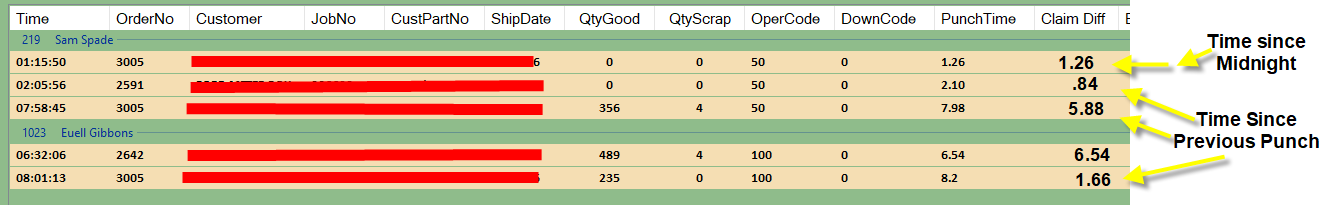asked on

# Calculated Values in VB ListView Groupings

I am using a ListView to view data from a MySqlDB.

ListView name is lstMySqlData -- I am able to pull in the data from MySql into the ListView -- then sort into Groups using the Sub code below by calling sub using the following: GroupByEmpNo(lstMySqlData, 1)

See resulting screen shot below:

My question is how to calculate the Claim Diff field by group as shown. The first row of each group would be the time in 100ths since midnight. Each row after that would be the amount of time since the last punch.

Thoughts -- example code -- other

Dan``````    Public Sub GroupByEmpNo(ByVal lstV As System.Windows.Forms.ListView, ByVal SubItemIndex As Int16)        Dim flag As Boolean = True
For Each l As ListViewItem In lstV.Items
Dim strmyGroupname As String = l.SubItems(SubItemIndex).Text
For Each lvg As ListViewGroup In lstV.Groups
If lvg.Name = strmyGroupname Then
l.Group = lvg
flag = False
End If
Next

Dim BackColor
BackColor = "FDF5E6"

For Each g As ListViewGroup In lstV.Groups
With g
For Each i As ListViewItem In .Items
i.Font = New Font("Calibri", 10, FontStyle.Bold)
i.BackColor = Color.Wheat
Next
End With
Next
If flag = True Then
Dim lstGrp As New ListViewGroup(strmyGroupname, strmyGroupname)
l.Group = lstGrp
End If
flag = True
Next
End Sub
``````
Visual Basic.NETLast Comment
louisfr

8/22/2022 - Mon